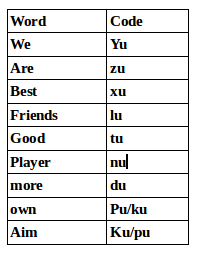## Coding and Decoding For SBI PO : Set – 41

D.1-5) Study the following and answer the questions

In a certain code language

” kite fly in sit ” is coded as ” X25G D5L S20T M14J “

“exam date are search” is coded as ” L13F D5B D5E G8T”

” solution is must for” is coded as” M14T S20N R19J Q18G”

” very problem may wrong” is coded as ” F7X X25N L13Q X25W”

FLY -> X 25 G

F -> G Immediate succeeding letter

L -> X Just Preceding Letter

Y -> 25 Numerical value of last letter

IN -> M 14 J

I -> J Immediate succeeding letter

N -> 14 Numerical Value of Last letter

N -> M Just Preceding Letter

1) What is the code for ‘school’ in the given code language?

(a) K21R

(b) R12K

(c) K12T

(d) K34R

(e)None of these

c)

K12T

2) What is the code for ‘fight problem’ in the given code language?

(a) L13Q S19G

(b) G20SL13Q

(c) S20GL13Q

(d) L13Q S19T

(e)None of these

c)S20G L13Q

3) What may be the possible code for ‘money quick sky ’ in the given code language?

(a) X25N J11R T25X

(b) J11R X25T N25X

(c) J11S X25U N25X

(d) X25N X25T J11R

(e)None of these

d)X25N X25T J11R

4) What may be the possible code for ‘student’ in the given code language?

(a) T21S

(b) S20T

(c) S19U

(d) T20N

(e)None of these

b)S20T

5) What is the code for ‘Kind Work’ in the given code language?

(a) L12X L4C

(b) K12X L4C

(c) J11X C4L

(d) L11X L4C

(e)None of these

c)J11X C4L

In a certain code ‘we are best friends’ is written as ‘xu zu lu yu’ ‘we good are player’ is written as ‘tu yu nu zu’, ‘friends best good more’ is written as ‘tu xu du lu’ and ‘are best own aim’ is written as ‘pu xu ku zu’.6) What is the code for ‘more’?

(a) tu

(b) xu

(c) du

(d) lu

(e) Cannot be determined

c)

du

7) What is the code for ‘friends’?

(a) lu

(b) xu

(c) du

(d) tu

(e) du or zu

a)

lu

8) Which of the following may represent ‘more player are aim’?

(a) nu zu pu ku

(b) ku nu zu yu

(c) du pu ku zu

(d) nu du pu zu

(e) du pu ku xu

d)

nu du pu zu

9) What does ‘nu’ stand for?

(a) player

(b) we

(c) good

(d) are

(e) Cannot be determined

a)

player

10) Which of the following may represent ‘own are good player’?

(a) ku tu zu pu

(b) pu du zu nu

(c) xu nu tu ku

(d) tu zu nu xu

(e) tu nu zu ku